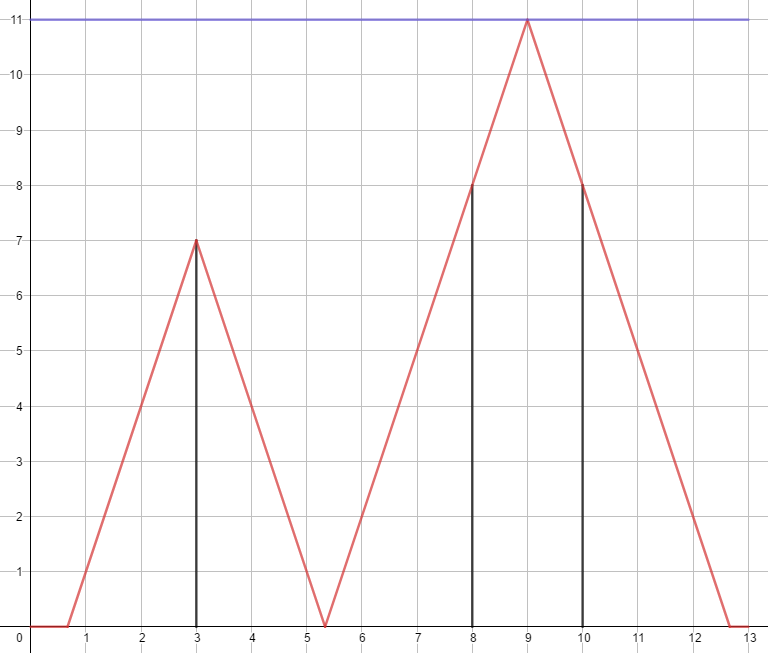High Jump
Tag(s):

Problem
Editorial
Analytics

There's a line of $L$ meters. You need to start at $0$, and arrive $L$. There are $N$ obstacles on the line. Each obstacle has a position $p_i$ and height $h_i$.

You have two ways to move, first one - just walk towards the destination, second one - jump to avoid obstacles.

If you are $X$ meters far from the start point, and $Y$ meters high, we say you are at $(X,Y)$. A jump means, start from $(X,0)$, travel along the line $(X,0)-(X+\frac{Y}{A},Y)$ and then $(X+\frac{Y}{A},Y)-(X+\frac{2Y}{A},0)$, which $(X,0)$ is the start position of the jump, and $Y$ is the height of the jump. If one of the two lines pass through any obstacle, this jump is illegal (It's legal to pass through the highest point of a obstacle). The height $Y$ is decide by yourself, but it should less than $M$. $A$ is your rising speed and falling speed.

Arrive $L$ means, your position should be (L,0) at some time.

You need to determine if it's possible to arrive $L$.

Input Format

First line contains four integers, $N,M,L,A(1\le N\le 10^5,1\le M,L,A\le 10^9)$.

The $(i+1)$ th line contains two integers, $p_i,h_i(1\le p_i<L,1\le h_i\le 10^9)$.

Output Format

"Yes" or "No".

SAMPLE INPUT
3 11 13 3
3 7
8 8
10 8

SAMPLE OUTPUT
Yes
Explanation

Check this graph. The red line is your path, the blue line is the height limit, and the black lines are the obstacles. In this sample, if $M=10$, the result will be "No".Time Limit: 1.0 sec(s) for each input file.
Memory Limit: 256 MB
Source Limit: 1024 KB
Marking Scheme: Marks are awarded when all the testcases pass.
Allowed Languages: Bash, C, C++, C++14, Clojure, C#, D, Erlang, F#, Go, Groovy, Haskell, Java, Java 8, JavaScript(Rhino), JavaScript(Node.js), Julia, Kotlin, Lisp, Lisp (SBCL), Lua, Objective-C, OCaml, Octave, Pascal, Perl, PHP, Python, Python 3, R(RScript), Racket, Ruby, Rust, Scala, Swift, Swift-4.1, TypeScript, Visual Basic

CODE EDITOR

Initializing Code Editor...

ContributorChallenge Name

September Circuits '18

OTHER PROBLEMS OF THIS CHALLENGE
• Basic Programming > Implementation
• Math > Convex Hull
• Basic Programming > Recursion
• Algorithms > Dynamic Programming
• Data Structures > Trees